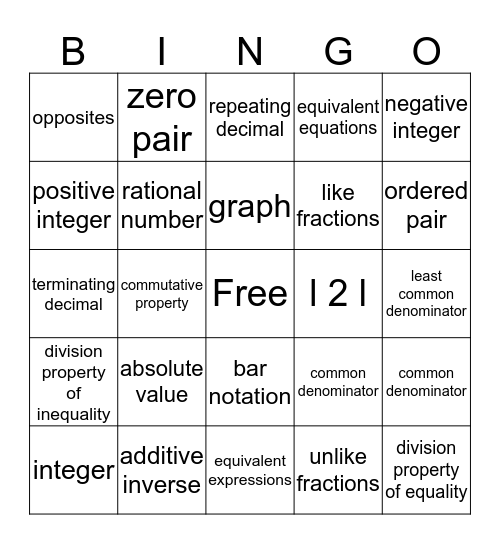# Chapters 3 & 4 Math VocabularyThis bingo card has a free space and 24 words: absolute value, additive inverse, graph, integer, negative integer, opposites, positive integer, zero pair, l 2 l, bar notation, like fractions, terminating decimal, common denominator, common denominator, rational number, unlike fractions, least common denominator, repeating decimal, ordered pair, commutative property, division property of equality, division property of inequality, equivalent equations and equivalent expressions.

⚠ This card has duplicate items: common denominator (2)

## Play Online